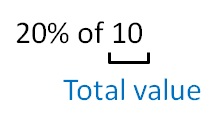Using percentage

Chapter 7 Class 7 Comparing Quantities
Concept wise

Sometimes we are given statements like

20% of 10

or

80% of 400

But what does that mean?Here, 10 is the total value

& we have to find 20% of it

How do we find it?

We replace ‘of’ with ×

20%  of 10 = 20% × 10

= 20/100 × 10

= 2/10 × 10

= 2

Similarly,

80%  of 400 = 80% × 400

= 80/100 × 400

= 80/1 × 4

= 320

Learn in your speed, with individual attention - Teachoo Maths 1-on-1 Class